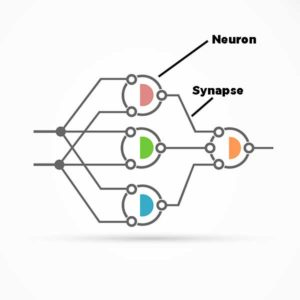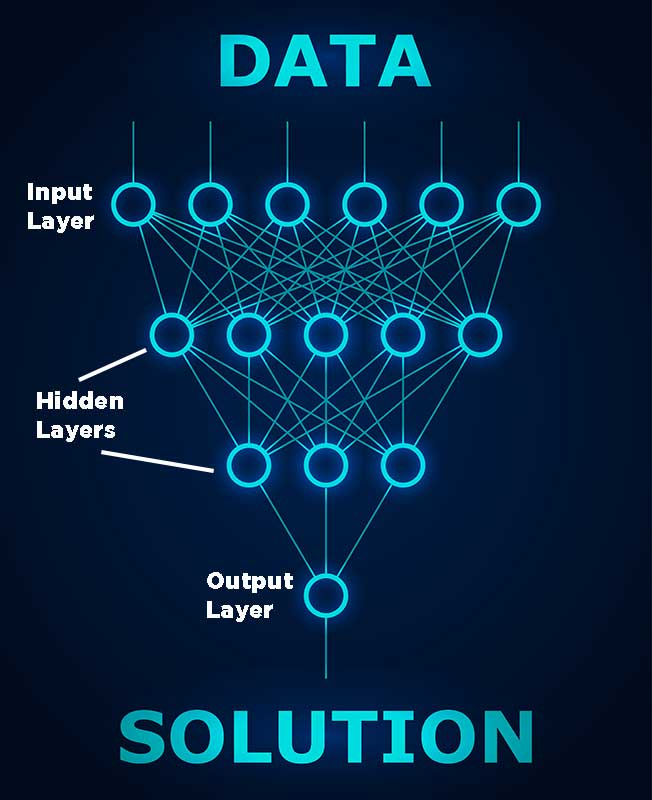## Getting Deeper with Deep Learning: Part 1

In my previous post, I introduced AI, machine learning, and deep learning. Now we are going to follow the white hare further down the AI rabbit hole and get more into machine learning, while also beginning our foray into Deep Learning. I’ll explain the basic structure of neural networks, of what they are comprised, and some general guidelines on how to create the optimal neural network for the dataset you will be processing. All references to neural networks in this post are references to artificial neural networks.Neural networks are based on a collection of units called artificial neurons. If you are familiar with the biological brain, artificial neurons are more analogous to axons (the protrusion from the biological neuron that conducts the electrical impulses) than actual neurons. The connections between the artificial neurons are called synapses and transmit the signal from neuron to neuron. Neurons are usually organized into layers in a neural network.

The first layer is always the input layer and consists of a number of neurons equal to the number of columns in your data set (also known as “features” when speaking in terms of machine learning) and sometimes one additional node for a bias term. The bias term is typically used to centralize your data if it is not already centralized. Think about this in mathematical terms: when you need to shift your algorithm to go through the 0, 0 point on a graph. The bias term is where you exercise this shift.

The last layer is always the output layer and the number of neurons in this layer is dependent upon the goal of your specific neural network. In general, neural networks are used for unsupervised learning (grouping unlabeled data), classification (categorization of the data), or regression (predicting continuous values after supervised training). The first two usually result in having multiple nodes in the output layer of a neural network with the number of nodes being the number of groups or categories where you want the data. With the regression method, you have (most commonly) only one node in the output layer that contains the value. I have frequently seen the regression method described in terms of property value in real estate. If you have w number of bedrooms, x square feet, and y lot size, that property is worth z number of dollars.The hidden layers are the layers between the input layer and the output layer and a neural network can vary between 0 and unlimited hidden layers. Granted, a single hidden layer is usually sufficient for a large majority of problems in which a neural network would be used. The number of neurons in the hidden layers can vary, but the general rule of thumb is that the neuron count in the hidden layers is the mean of the neuron count in the input layer plus the neuron count in the output layer (7 columns of data for input, plus 1 neuron that will contain the result, has a mean of 4 neurons in the hidden layer (7 + 1 = 8 / 2 = 4).

The best way to find the optimal network configuration for the number of nodes (neurons) in your hidden layers lies in applying a pruning algorithm that will remove nodes which, if removed from the neural network, would not have a noticeable effect on network performance. This mechanism looks at the weight matrix and removes nodes that have a weight very close to zero.

I realize this is a fair amount of information to digest, but if you’re not thoroughly confused at this point (and hopefully you’re not) stay tuned to BOXX Blogs as we continue this informative series with Getting Deeper with Deep Learning Part II.
​_______________________
Previous Post in the Deep Learning series: Introduction to Deep Learning
Next post in the Deep Learning series: Getting Deeper with Deep Learning: Part 2